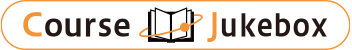### CourseDetailDegree
Bachelor
Course delivery methods
face-to-face
Subject
Engineering & technology
Program
School
Engineering School (EP)
Department
Campus
São Paulo main campus
Classroom
Course Offering Year
Course Offering Month
January - January
Weekday and Period
Capacity
32
Credits
8
Language
English
Course Number
PMR5412

### Modelling and Numerical Simulation via Variational Calculus University of Sao Paulo

#### Course Overview

The finite element computational simulation methodology, starting with the variational formulation of the problem, allows students to quickly familiarize themselves with finite element theory, to solve complex partial differential equations, to implement their own customized software to simulate a specific physical phenomenon required in their research, and to learn the necessity and importance of the variational calculus. It is a different approach in relation to what is present in the current finite element computational simulation commercial software, which contains the implementation of the final discrete (matrix) formulation of a problem, thus limiting the physical phenomena (differential equations) that can be modeled only to those problems already implemented in the Software. In the new approach we start with the variational formulation, thus allowing more flexibility and generality in the computational simulation of the physical phenomena (partial differential equations).

#### Learning Achievement

The aim of this course is to teach the concepts of a finite element computational simulation methodology that allows starting from the variational formulation of the problem to solve partial differential equations. In this way, concepts will be explored on the finite element method, variational calculation, high order linear system solution techniques and other concepts involved in the method. As a tool will be used FENICS software (free license), which is a recent computational tool that follows the concept of modeling from the variational formulation.

#### Course prerequisites

Based on Project (10-page report)

#### Course schedule

1. Introduction to the finite element method (Implementation of the Poisson equation, Verifying code with the method of manufactured solutions) 2. Constructive solid geometry; Geometric singularities in the L-shaped domain 3. Adding a potential term; Varying the type of boundary conditions; Integrating over subdomains 4. The Newton-Kantorovich method for PDEs 5. Nonlinear radiation boundary conditions 6. Continuation for nonlinear problems 7. Solution of time-dependent PDEs (PDEs on manifolds) 8. Mixed variational problems: the Stokes equations 9. Nonlinear hyperelasticity 10. Variational inequalities with semismooth Newton methods 11. Eigenvalue problems 12. Oneshot methods for PDE-constrained optimisation problems 13. Parallelism in PDE solvers with MPI 14. Algebraic and geometric multigrid methods 15. Schur complement preconditioners: the Stokes equations 16. Bifurcation analysis of PDEs 17. Bifurcation analysis of the Navier-Stokes equations

#### Instructor

Emilio Carlos Nelli Silva